TitleIntermediate Algebra
Answer/Discussion to Practice Problems
Tutorial 4: Operations on NumbersAnswer/Discussion to 1a  -5 + (-16)   -5 + (-16) = -21.  Note that we are adding two numbers with the same sign.  The sum of the absolute values 5 and 16 is 21 and their common sign is negative. Therefore, our answer is -21. (return to problem 1a)Answer/Discussion to 1b 7 + (-3)   7 + (-3) = 4.  Note that we are adding two numbers that have opposite signs.  The difference between the absolute values of 7 and 3 is 4 and the sign of the number with the larger absolute value (7) is positive.  Therefore, our answer is 4. (return to problem 1b)Answer/Discussion to 1c  -10 - (-2) -10 - (-2) = -10 + 2 = -8.  Subtracting a negative 2 is the same as adding a positive 2.  Once we change it to addition, we now are adding two numbers with opposite signs.  The difference of the absolute values of 10 and 2 is 8 and the sign of the larger absolute value (-10) is negative.  Therefore, our answer is -8. (return to problem 1c)Answer/Discussion to 2a  (-4)(9)    (-4)(9) = -36.  We are multiplying two numbers with opposite signs.  The product of 4 and 9 is 36 and multiplying with opposite signs gives us a negative. Therefore, our answer is -36. (return to problem 2a)Answer/Discussion to 2b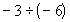= .5 or ½.  We are dividing two numbers with the same sign.  The quotient of 3 and 6 is .5 and dividing with the same signs gives us a positive number. Therefore, our answer is .5. (return to problem 2b)Answer/Discussion to 3a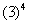*Write the base 3 in a product 4 times *MultiplyAnswer/Discussion to 3b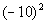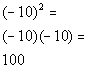*Write the base -10 in a product 2 times *MultiplyAnswer/Discussion to 3c*Negate 10 squared *Put a - in front of 10 written in a product 2 times *MultiplyAnswer/Discussion to 4a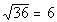The thought behind this is that we are looking for the square root of 36, which means we are looking for a number that when we square it we get 36.   Since 6 squared is 36, 6 is the square root of 36.  Note that we are only interested in the principal root and since 36 is positive and there is not a sign in front of the radical, our answer is positive 6.  If there had been a negative in front of the radical our answer would have been -6Answer/Discussion to 4b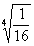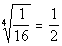Now we are looking for the fourth root of 1/16, which means we are looking for a number that when we take it to the 4th power, we get 1/16.  Since 1/2 to the 4th power is 1/16, our answer is going to be 1/2. (return to problem 4b)Answer/Discussion to 5a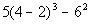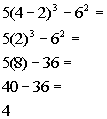*(  )  *Exponents *Multiplication *SubtractionAnswer/Discussion to 5b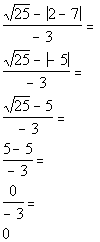*Absolute value  *(fancy grouping symbol) *Square root    *Subtraction in numerator *DivideAnswer/Discussion to 6a Find the value of the expression when a = 3 and b = -2.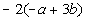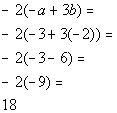*Replace a with 3 and b with -2 *Multiply within (  ) *Subtract within (  ) *Multiply

Last revised on June 10, 2011 by Kim Seward.
All contents copyright (C) 2002 - 2011, WTAMU and Kim Seward. All rights reserved.